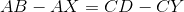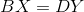# ISEE Upper Level Quantitative : How to find the length of the side of a rectangle

## Example Questions

### Example Question #1 : How To Find The Length Of The Side Of A Rectangle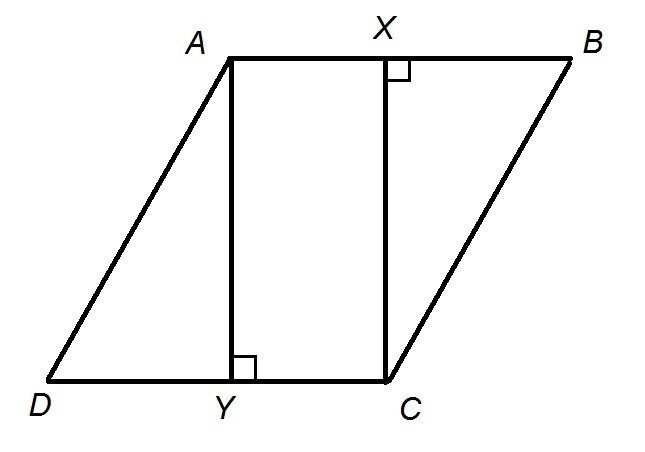Note: Figure NOT drawn to scale

The above figure shows Rhombus.

Which is the greater quantity?

(a)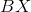(b)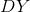It is impossible to determine which is greater from the information given

(a) and (b) are equal

(b) is the greater quantity

(a) is the greater quantity

(a) and (b) are equal

Explanation:

The opposite sides of a parallelogram - a rhombus included - are congruent, so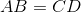.

Also, Quadrilateral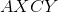form a rectangle; since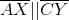and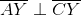, it follows that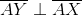, and, similarly,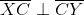. Therefore,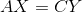, and